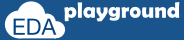# unique if

Unique if evaluates all the conditions parallel.

In the following conditions simulator issue a run time error/warning,
• More than one condition is true
• No condition is true or final if doesn't have corresponding else

## Unique if Example-1:

In the below example,
More than one condition is true.
value of a=10, b=20 and c=40. conditions a<b and a<c are true,

Therefore on execution, simulator issue a run time warning.
"RT Warning: More than one condition match in 'unique if' statement."

```module unique_if;
```
```  //variables declaration
int a,b,c;

initial begin
//initialization
a=10;
b=20;
c=40;

unique if ( a < b ) \$display("\t a is less than b");
else   if ( a < c ) \$display("\t a is less than c");
else                \$display("\t a is greater than b and c");
end
endmodule```

Simulator output:

a is less than b
RT Warning: More than one conditions match in 'unique if' statement.

Execute the above code onExample-2:

In below example,
No condition is true and final if doesn't have corresponding else.
value of a=50, b=20 and c=40, conditions a<b and a<c are false,

Therefore on execution, simulator issue a run time warning.
"RT Warning: No condition matches in 'unique if' statement."

```module unique_if;
```
```  //variables declaration
int a,b,c;

initial begin
//initialization
a=50;
b=20;
c=40;

unique if ( a < b ) \$display("\t a is less than b");
else   if ( a < c ) \$display("\t a is less than c");
end

endmodule```

Simulator output:

RT Warning: No condition matches in 'unique if' statement

Execute the above code onExample-3:

In below example, value of a=50, b=20 and c=40.
conditions a<b and a<c are false, so else part is true, there is no simulator run time warning.

```module unique_if;

//variables declaration
int a,b,c;

initial begin
//initialization
a=50;
b=20;
c=40;

priority if ( a < b ) \$display("\t a is less than b");
else     if ( a < c ) \$display("\t a is less than c");
else                  \$display("\t a is greater than b and c");
end

endmodule```

Simulator output:

a is greater than b and c

Execute the above code on